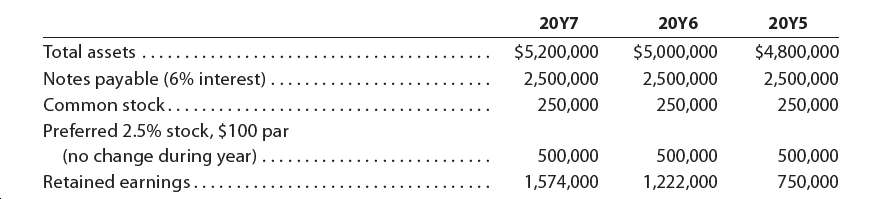# 20Y720Υ620Y5Total assets ..\$5,200,000\$5,000,000\$4,800,000Notes payable (6% interest) .Common stock......Preferred 2.5% stock, \$100 par(no change during year)Retained earnings.....2,500,0002,500,0002,500,000250,000250,000250,000500,000500,000500,0001,574,0001,222,000750,000

Question
6 views

The following selected data were taken from the financial statements of Vidahill Inc. for December 31, 20Y7, 20Y6, and 20Y5:

Please see  the attachment for details:

The 20Y7 net income was \$411,000, and the 20Y6 net income was \$462,500. No dividends on common stock were declared between 20Y5 and 20Y7. Preferred dividends were declared and paid in full in 20Y6 and 20Y7.
a. Determine the return on total assets, the return on stockholders’ equity, and the return on common stockholders’ equity for the years 20Y6 and 20Y7. Round percentages to one decimal place.
b. What conclusions can be drawn from these data as to the company’s
profitability?help_outlineImage Transcriptionclose20Y7 20Υ6 20Y5 Total assets .. \$5,200,000 \$5,000,000 \$4,800,000 Notes payable (6% interest) . Common stock...... Preferred 2.5% stock, \$100 par (no change during year) Retained earnings..... 2,500,000 2,500,000 2,500,000 250,000 250,000 250,000 500,000 500,000 500,000 1,574,000 1,222,000 750,000 fullscreen
check_circle

Step 1

Hence, the return on total asset in 2017 was 11%. It is calculated by dividing the sum of net income and interest on notes payable by the average total assets. The net income is \$411,000 and interest on notes payable is calculated by multiplying notes payable worth \$2,500,000 by interest rate of 6%. The average total assets is calculated by dividing the sum of opening total assets worth \$5,000,000 and closing total assets worth \$5,200,000 by 2.

Step 2

Hence, the return on total asset in 2016 was 12.5%. It is calculated by dividing the sum of net income and interest on notes payable by the average total assets. The net income is \$462,500 and interest on notes payable is calculated by multiplying notes payable worth \$2,500,000 by interest rate of 6%. The average total assets is calculated by dividing the sum of opening total assets worth \$4,800,000 and closing total assets worth \$5,000,000 by 2.

Step 3

Hence, the return on stockholder’s equity in 2017 was 19.1%. It is calculated by dividing the net income by the average stockholder’s equity. The net income is \$411,000. The average stockholder’s equity is calculated by dividing...

### Want to see the full answer?

See Solution

#### Want to see this answer and more?

Solutions are written by subject experts who are available 24/7. Questions are typically answered within 1 hour.*

See Solution
*Response times may vary by subject and question.
Tagged in